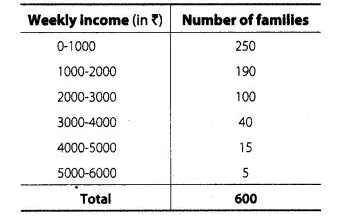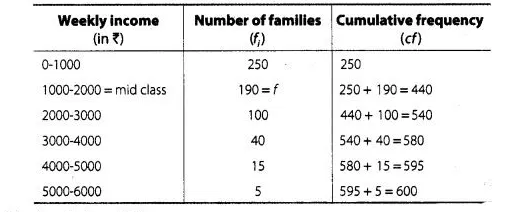# Weekly income of 600 families is tabulated below

Question:

Weekly income of 600 families is tabulated belowCompute the median income.

Solution:

First we construct a cumulative frequency tableIt is given that, $n=600$

$\therefore$ $\frac{n}{2}=\frac{600}{2}=300$

Since, cumulative frequency 440 lies in the interval $1000-2000$.

Here, (lower median class) $l=1000$,

$f=190, c f=250$, (class width) $h=1000$

and (total observation) $n=600$

$\therefore$ Median $=l+\frac{\left\{\frac{n}{2}-c f\right\}}{f} \times n$

$=1000+\frac{(300-250)}{190} \times 1000$

$=1000+\frac{50}{190} \times 1000$

$=1000+\frac{5000}{19}$

$=1000+263.15=1263.15$

Hence, the median income is  ₹ 1263.15.